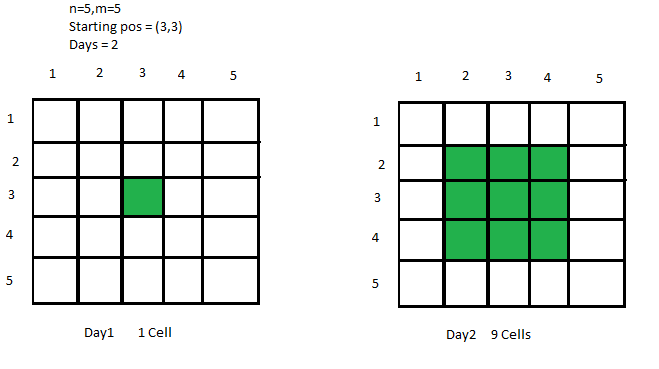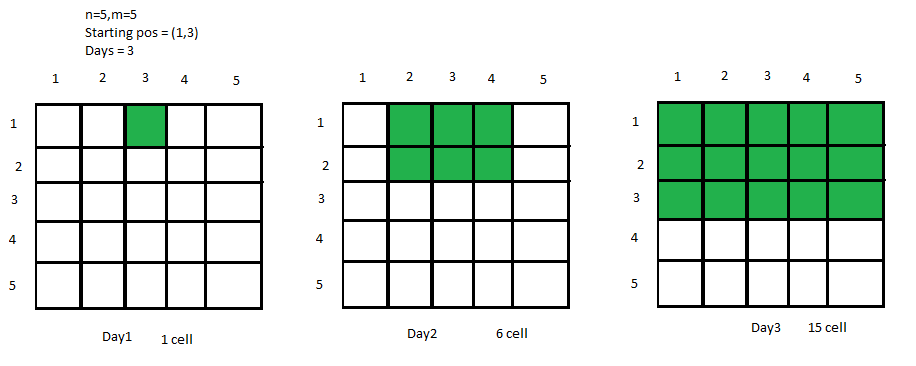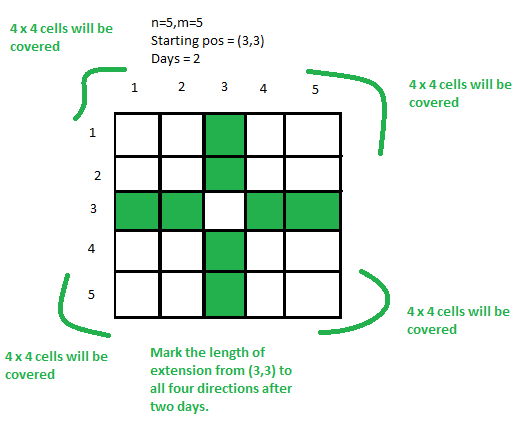GeeksforGeeks App
Open AppBrowser
Continue

# Total number of cells covered in a matrix after D days

Given an N * M matrix and a starting position (X, Y) of a virus, the task is to find out the number of covered cells after D days, if the virus spreads from its current cell to its adjacent cells every day.Examples:

Input: N = 5, M = 5, X = 1, Y = 3, D = 3Output: 15
Explanation:
We can clearly see from the picture that 15 cells are covered after 3 days.
Input: N = 10, M = 10, X = 7, Y = 8, D = 4
Output: 42
Explanation:
On making an N * M matrix and filling the adjacent cells for 4 days we will get 42 covered cells.

Approach:
To solve the problem mentioned above we have to observe clearly that from a starting cell, we just need to find out the extension of cells towards top, right, bottom and left after D days. Then calculate the total cells inside every quadrilateral of cells formed and add them all.
Therefore, the total answer will be the sum of all cells of quadrilaterals after D days + the total cells that are along the top, right, down, left, and 1 (for Starting cell) keeping in consideration the boundaries of the quadrilateral.
Below is the condition for extension in all four directions:

Extension upto Top -> min(D, X-1)
Extension upto Down -> min(D, N-X)
Extension upto Left -> min(D, Y-1)
Extension upto Right -> min(D, M-Y)

Look at the image below for clear understanding:Now multiply Top * Left, Top * Right, Down * Left, Down * Right and add all of them and also add the total cells along the line of 4 directions. We also add 1(for starting cell) to get the resultant cells.
Below is the implementation of above approach:

## C++

 `// C++ implementation to find the``// Total number of cells covered``// in a matrix after D days` `#include ``using` `namespace` `std;` `// Function to return the total``// infected cells after d days``int` `solve(``int` `n, ``int` `m, ``int` `x, ``int` `y, ``int` `d)``{``    ``// Top extension``    ``int` `top = min(d, x - 1);` `    ``// Bottom extension``    ``int` `down = min(d, n - x);` `    ``// Left extension``    ``int` `left = min(d, y - 1);` `    ``// Right extension``    ``int` `right = min(d, m - y);` `    ``// Calculating the cells``    ``// in each quadrilateral``    ``int` `quad1 = top * left;``    ``int` `quad2 = left * down;``    ``int` `quad3 = down * right;``    ``int` `quad4 = right * top;` `    ``// Sum all of them to get``    ``// total cells in each``    ``// quadrilateral``    ``int` `totalsq = quad1 + quad2 + quad3 + quad4;` `    ``// Add the singleblocks``    ``// along the lines of top,``    ``// down, left, right``    ``int` `singleBlocks = top + down + left + right + 1;` `    ``// Return the ans``    ``return` `totalsq + singleBlocks;``}` `// Driver code``int` `main()``{``    ``int` `n, m, x, y, d;` `    ``// Dimensions of cell``    ``n = 10, m = 10;` `    ``// Starting Coordinates``    ``x = 7, y = 8;` `    ``// Number of Days``    ``d = 4;``    ``d--;` `    ``// Function Call``    ``cout << solve(n, m, x, y, d);``}`

## Java

 `// Java implementation to find the``// total number of cells covered``// in a matrix after D days``import` `java.util.*;` `class` `GFG {` `    ``// Function to return the total``    ``// infected cells after d days``    ``static` `int` `solve(``int` `n, ``int` `m, ``int` `x, ``int` `y, ``int` `d)``    ``{` `        ``// Top extension``        ``int` `top = Math.min(d, x - ``1``);` `        ``// Bottom extension``        ``int` `down = Math.min(d, n - x);` `        ``// Left extension``        ``int` `left = Math.min(d, y - ``1``);` `        ``// Right extension``        ``int` `right = Math.min(d, m - y);` `        ``// Calculating the cells``        ``// in each quadrilateral``        ``int` `quad1 = top * left;``        ``int` `quad2 = left * down;``        ``int` `quad3 = down * right;``        ``int` `quad4 = right * top;` `        ``// Sum all of them to get``        ``// total cells in each``        ``// quadrilateral``        ``int` `totalsq = quad1 + quad2 + quad3 + quad4;` `        ``// Add the singleblocks``        ``// along the lines of top,``        ``// down, left, right``        ``int` `singleBlocks = top + down + left + right + ``1``;` `        ``// Return the ans``        ``return` `totalsq + singleBlocks;``    ``}` `    ``// Driver code``    ``public` `static` `void` `main(String[] args)``    ``{` `        ``// Dimensions of cell``        ``int` `n = ``10``, m = ``10``;` `        ``// Starting coordinates``        ``int` `x = ``7``, y = ``8``;` `        ``// Number of days``        ``int` `d = ``4``;``        ``d--;` `        ``// Function call``        ``System.out.println(solve(n, m, x, y, d));``    ``}``}` `// This code is contributed by Pratima Pandey`

## Python3

 `# Python3 implementation to find the``# total number of cells covered in``# a matrix after D days` `# Function to return the total``# infected cells after d days`  `def` `solve(n, m, x, y, d):` `    ``# Top extension``    ``top ``=` `min``(d, x ``-` `1``)` `    ``# Bottom extension``    ``down ``=` `min``(d, n ``-` `x)` `    ``# Left extension``    ``left ``=` `min``(d, y ``-` `1``)` `    ``# Right extension``    ``right ``=` `min``(d, m ``-` `y)` `    ``# Calculating the cells``    ``# in each quadrilateral``    ``quad1 ``=` `top ``*` `left``    ``quad2 ``=` `left ``*` `down``    ``quad3 ``=` `down ``*` `right``    ``quad4 ``=` `right ``*` `top` `    ``# Sum all of them to get``    ``# total cells in each``    ``# quadrilateral``    ``totalsq ``=` `(quad1 ``+` `quad2 ``+``               ``quad3 ``+` `quad4)` `    ``# Add the singleblocks``    ``# along the lines of top,``    ``# down, left, right``    ``singleBlocks ``=` `(top ``+` `down ``+``                    ``left ``+` `right ``+` `1``)` `    ``# Return the ans``    ``return` `totalsq ``+` `singleBlocks`  `# Driver Code``if` `__name__ ``=``=` `'__main__'``:` `    ``# Dimensions of cell``    ``n ``=` `10``    ``m ``=` `10` `    ``# Starting Coordinates``    ``x ``=` `7``    ``y ``=` `8` `    ``# Number of Days``    ``d ``=` `4``    ``d ``-``=` `1` `    ``# Function Call``    ``print``(solve(n, m, x, y, d))` `# This code is contributed by Shivam Singh`

## C#

 `// C# implementation to find the``// total number of cells covered``// in a matrix after D days``using` `System;``class` `GFG {` `    ``// Function to return the total``    ``// infected cells after d days``    ``static` `int` `solve(``int` `n, ``int` `m, ``int` `x, ``int` `y, ``int` `d)``    ``{` `        ``// Top extension``        ``int` `top = Math.Min(d, x - 1);` `        ``// Bottom extension``        ``int` `down = Math.Min(d, n - x);` `        ``// Left extension``        ``int` `left = Math.Min(d, y - 1);` `        ``// Right extension``        ``int` `right = Math.Min(d, m - y);` `        ``// Calculating the cells``        ``// in each quadrilateral``        ``int` `quad1 = top * left;``        ``int` `quad2 = left * down;``        ``int` `quad3 = down * right;``        ``int` `quad4 = right * top;` `        ``// Sum all of them to get``        ``// total cells in each``        ``// quadrilateral``        ``int` `totalsq = quad1 + quad2 + quad3 + quad4;` `        ``// Add the singleblocks``        ``// along the lines of top,``        ``// down, left, right``        ``int` `singleBlocks = top + down + left + right + 1;` `        ``// Return the ans``        ``return` `totalsq + singleBlocks;``    ``}` `    ``// Driver code``    ``public` `static` `void` `Main(String[] args)``    ``{` `        ``// Dimensions of cell``        ``int` `n = 10, m = 10;` `        ``// Starting coordinates``        ``int` `x = 7, y = 8;` `        ``// Number of days``        ``int` `d = 4;``        ``d--;` `        ``// Function call``        ``Console.WriteLine(solve(n, m, x, y, d));``    ``}``}` `// This code is contributed by Rohit_ranjan`

## Javascript

 ``

Output:

`42`

Time Complexity: O(1)
Auxiliary Space: O(1)

My Personal Notes arrow_drop_up